# Solution assignment 06 Exponential functions and graphs

### Assignment 6

Draw the graph of the function: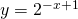What is the formula of the asymptote?

### Solution

We can draw the graph of the function by calculating some characteristic points. First, the point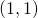. This is because the graph of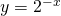has shifted byto the right.

For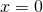we find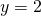. We know that the graph will approach the-axis for increasing(the-axis is the asymptote), and thus we can draw the graph, see the figure.0